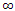# Electronics - Field Effect Transistors (FET)

### Exercise :: Field Effect Transistors (FET) - General Questions

6.

When testing an n-channel D-MOSFET, resistance G to D =, resistance G to S =, resistance D to SS =and 500, depending on the polarity of the ohmmeter, and resistance D to S = 500. What is wrong?

 A. short D to S B. open G to D C. open D to SS D. nothing

Explanation:

No answer description available for this question. Let us discuss.

7.

In the constant-current region, how will the IDS change in an n-channel JFET?

 A. As VGS decreases ID decreases. B. As VGS increases ID increases. C. As VGS decreases ID remains constant. D. As VGS increases ID remains constant.

Explanation:

No answer description available for this question. Let us discuss.

8.

A MOSFET has how many terminals?

 A. 2 or 3 B. 3 C. 4 D. 3 or 4

Explanation:

No answer description available for this question. Let us discuss.

9.

IDSS can be defined as:

 A. the minimum possible drain current B. the maximum possible current with VGS held at –4 V C. the maximum possible current with VGS held at 0 V D. the maximum drain current with the source shorted

Explanation:

No answer description available for this question. Let us discuss.

10.

What is the input impedance of a common-gate configured JFET?

 A. very low B. low C. high D. very high